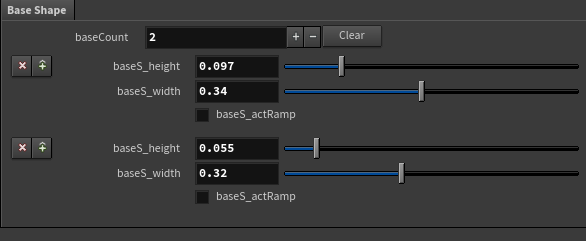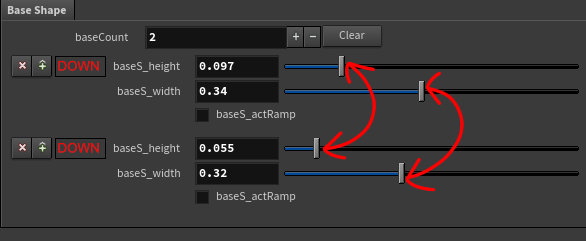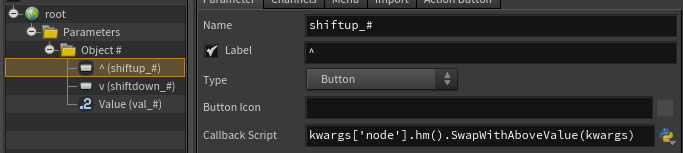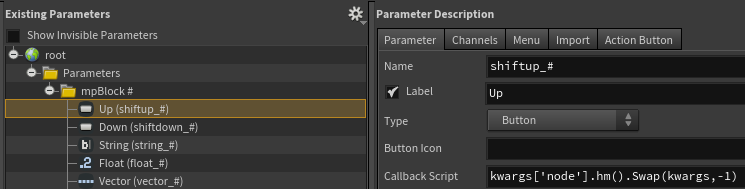## Recommended Posts

Hi everyone,

Is it possible to reorder easily a Multiparm Block list?(without deleting and recreating the exact value)

I would love to swipe the order easily if I have many number of item. (ex: swipe the first and the second item on my reference)

Cheers##### Share on other sites

Maybe someone has a python script for a button to swap values with the next parameter block?##### Share on other sites

@konstantin magnus As per your suggestion, I wrote a simple python script that swaps a multi-parameter's value with one before/after it.

```import re
def SwapWithAboveValue(kwargs):

node = kwargs['node']
params = node.parm("parms").evalAsInt()
self = kwargs["parm_name"]
index = int(re.findall(r'\d+', self))

if params > 1:
valueSelf = node.parm("val_%s" % int(index)).evalAsFloat()
valueAbove = node.parm("val_%s" % int(index-1)).evalAsFloat()
node.parm("val_%s" % int(index-1)).set(valueSelf)
node.parm("val_%s" % int(index)).set(valueAbove)

def SwapWithBelowValue(kwargs):

node = kwargs['node']
params = node.parm("parms").evalAsInt()
self = kwargs["parm_name"]
index = int(re.findall(r'\d+', self))

if params > 1:
valueSelf = node.parm("val_%s" % int(index)).evalAsFloat()
valueBelow = node.parm("val_%s" % int(index+1)).evalAsFloat()
node.parm("val_%s" % int(index+1)).set(valueSelf)
node.parm("val_%s" % int(index)).set(valueBelow)
```Edited by Fenolis
•1

##### Share on other sites

Thanks alot

I have created a digital asset, pasted your code as Python module in 'Scripts' and inserted the callback scripts as shown. Unfortunately when clicking on up or down, it's throwing an error:

```Traceback (most recent call last):
File "Sop/konst_csg/shift_down2", line 1, in <module>
File "Sop/konst_csg, PythonModule", line 18, in SwapWithBelowValue
AttributeError: 'NoneType' object has no attribute 'evalAsInt'```

Any idea what I might be missing?

##### Share on other sites

@konstantin magnus my Parameters (multiparm) folder is named "parms", which is what I'm using to check that there are at least 2 multiparm blocks in order for the buttons to have any use. I omitted the else portions of the if statements since I was trying to work out if it would be possible to use the Disable When field for better UX. I discovered that I could use a function like { isparm(shiftup_1) == 1 } to disable a parameter if it is in the first multiparm block, but couldn't find an equivalent solution for the last block.

The workaround I came up with makes a slight modification to throw a suitable error:

```def SwapWithBelowValue(kwargs):

node = kwargs['node']
params = node.parm("parms").evalAsInt()
self = kwargs["parm_name"]
index = int(re.findall(r'\d+', self))

if params > 1:
if index == params:
raise hou.NodeWarning("No value below to swap with.")
else:
valueSelf = node.parm("val_%s" % int(index)).evalAsFloat()
valueBelow = node.parm("val_%s" % int(index+1)).evalAsFloat()
node.parm("val_%s" % int(index+1)).set(valueSelf)
node.parm("val_%s" % int(index)).set(valueBelow)```

You can also modify the first function similarly for consistency, perhaps someone will figure out a more elegant solution that I missed.

##### Share on other sites

Also, this method only swaps single values. Expressions/channel references are not handled. I imagine it would be possible to store an array of values to switch with, but it could get complicated if you wish to switch nested multiparms, for instance. You would have to make sure the order in which values were swapped were correct - make sure multiparms have the right number of blocks before updating the values in each block. Perhaps some kind of check could be performed on the swappable parameter to determine its type and have the switching initialized based on that.

##### Share on other sites

When you are calling the function on the button, use -1 or 1 to swap up/down (technically you can swap with a value that's at any offset but for practical reasons...)

```#SwapValues(kwargs, hou.ParmTemplate.name a, hou.ParmTemplate.name b)
def SwapValues(kwargs, a, b):
#a, b are parameter names (not labels)
node = kwargs["node"]
pA = node.parm(a)
pB = node.parm(b)
if len(pA.keyframes()) == 0:
#if both params have no keyframes
if len(pB.keyframes()) == 0:
valueSelf = pA.rawValue()
valueOther = pB.rawValue()
pA.set(valueOther)
pB.set(valueSelf)
#if A has no keyframes but B does
else:
valueSelf = pA.rawValue()
valueOther = pB.keyframes()
pA.setKeyframes(valueOther)
pB.deleteAllKeyframes()
pB.set(valueSelf)
else:
#if A has keyframes but B doesn't
if len(pB.keyframes()) == 0:
valueSelf = pA.keyframes()
valueOther = pB.rawValue()
pA.deleteAllKeyframes()
pA.set(valueOther)
pB.setKeyframes(valueSelf)
#if both params have keyframes
else:
valueSelf = pA.keyframes()
valueOther = pB.keyframes()
pA.deleteAllKeyframes()
pB.deleteAllKeyframes()
pA.setKeyframes(valueOther)
pB.setKeyframes(valueSelf)

#GetParamNames(kwargs, hou.parmTemplate mpBlock, int index, int swapIndex, int nestingDepth)
def GetParamNames(kwargs, mpBlock, index, swapIndex, nestingDepth):
node = kwargs["node"]

for i in range(len(mpBlock)):
#If the current parameter is of a valid type, check if it has channels
if mpBlock[i].type() == hou.parmTemplateType.Int or mpBlock[i].type() == hou.parmTemplateType.Float or mpBlock[i].type() == hou.parmTemplateType.String or mpBlock[i].type() == hou.parmTemplateType.Toggle:

#note that vector channels are suffixed after multiparm index - "vector_#x" instead of "vector_x#"
if mpBlock[i].numComponents() > 1:
for c in range(mpBlock[i].numComponents()):
if mpBlock[i].namingScheme() == hou.parmNamingScheme.XYZW:
if c == 0:
vComponent = "x"
elif c == 1:
vComponent = "y"
elif c == 2:
vComponent = "z"
elif c == 3:
vComponent = "w"
elif mpBlock[i].namingScheme() == hou.parmNamingScheme.RGBA:
if c == 0:
vComponent = "r"
elif c == 1:
vComponent = "g"
elif c == 2:
vComponent = "b"
elif c == 3:
vComponent = "a"
elif mpBlock[i].namingScheme() == hou.parmNamingScheme.UVW:
if c == 0:
vComponent = "u"
elif c == 1:
vComponent = "v"
elif c == 2:
vComponent = "w"
pName = mpBlock[i].name().replace("#","%s") % index + vComponent
pOthrName = mpBlock[i].name().replace("#","%s") % (index+swapIndex) + vComponent

SwapValues(kwargs, pName, pOthrName)

else:
pName = mpBlock[i].name().replace("#","%s") % index
pOthrName = mpBlock[i].name().replace("#","%s") % (index+swapIndex)
SwapValues(kwargs, pName, pOthrName)

#if a folder is found, determine if it's a nested multiparm
elif mpBlock[i].type() == hou.parmTemplateType.Folder:
#if it is, compare the number of instances in each multiparm
if mpBlock[i].folderType() == hou.folderType.MultiparmBlock:

getNMP = mpBlock[i].name().replace("#","%s") % index
getOthrNMP = mpBlock[i].name().replace("#","%s") % (index+swapIndex)
nmpInstances = node.parm(getNMP).evalAsInt()
nmpOthrInstances = node.parm(getOthrNMP).evalAsInt()

#If both multiparms have the same number of instances, swap nested parameter values
if nmpInstances == nmpOthrInstances:
for j in range(nmpInstances):
pA = node.parm(getNMP).parmTemplate().parmTemplates()[j-1].name().replace("#","%s") % (index, j+1)
pB = node.parm(getOthrNMP).parmTemplate().parmTemplates()[j-1].name().replace("#","%s") % (index+swapIndex, j+1)
SwapValues(kwargs, pA, pB)
#Otherwise, save values to a temporary holder
else:
tempA = list()
tempB = list()
for j in range(nmpInstances):
nestedParm = node.parm(getNMP).parmTemplate().parmTemplates()[j-1].name().replace("#","%s") % (index, j+1)
if len(node.parm(nestedParm).keyframes()) > 0:
tempA.append(node.parm(nestedParm).keyframes())
else:
tempA.append(node.parm(nestedParm).rawValue())

for j in range(nmpOthrInstances):
nestedParm = node.parm(getOthrNMP).parmTemplate().parmTemplates()[j-1].name().replace("#","%s") % (index+swapIndex, j+1)
if len(node.parm(nestedParm).keyframes()) > 0:
tempB.append(node.parm(nestedParm).keyframes())
else:
tempB.append(node.parm(nestedParm).rawValue())

#initialize number of multiparm blocks
SwapValues(kwargs, getNMP, getOthrNMP)

#and update each block from the temporary holders
for k in range(nmpOthrInstances):
pA = node.parm(getNMP).parmTemplate().parmTemplates()[k-1].name().replace("#","%s") % (index, k+1)
node.parm(pA).deleteAllKeyframes()
try:
node.parm(pA).set(tempB[k])
except:
node.parm(pA).setKeyframes(tempB[k])
for k in range(nmpInstances):
pB = node.parm(getOthrNMP).parmTemplate().parmTemplates()[k-1].name().replace("#","%s") % (index+swapIndex, k+1)
node.parm(pB).deleteAllKeyframes()
try:
node.parm(pB).set(tempA[k])
except:
node.parm(pB).setKeyframes(tempA[k])

#if it's not a multiparm, dive inside and swap each nested parameter
else:
GetParamNames(kwargs, mpBlock[i].parmTemplates(), index, swapIndex, 0)

#Swap(kwargs, int targetSwapIndex)
def Swap(kwargs,targetSwapIndex):
node = kwargs["node"]
button = kwargs["parm"]

#Shorthand to access the index of a multiparm
index = int(kwargs["script_multiparm_index"])

#Raise error if parameter hierarchy is configured incorrectly
if not button.tuple().isMultiParmInstance():
raise hou.NodeWarning("Button is not inside a multiparm block.")

#Get the parent multiparm folder
mpFolder = button.tuple().parentMultiParm()

#Count the number of multiparm instances -> raise errors if swapping is not allowed
mpInstances = node.parm(mpFolder.name()).evalAsInt()

#Raise errors if trying to swap up on first block, or swap down on last block
if targetSwapIndex > 0:
if index == mpInstances:
raise hou.NodeWarning("No value below to swap with.")
elif targetSwapIndex < 0:
if index == 1:
raise hou.NodeWarning("No value above to swap with.")

#Get the other parameters inside this multiparm block so we can start swapping.
mpBlock = node.parm(mpFolder.name()).parmTemplate().parmTemplates()
GetParamNames(kwargs, mpBlock, index, targetSwapIndex, 0)```Edited by Fenolis
Added ability to swap certain types of nested multiparms at a depth of 1
•4

##### Share on other sites

@Fenolis: Thank you again! This is a much needed feature extension imho!

•1

##### Share on other sites

Swapping nested multiparms is definitely a recursive process, one that is on the verge of blowing my mind trying to think of how the nested parameter names are formatted. I've updated the snippet above to add the following features:
- Can swap multiple (not nested multiparm) parameters at once
- Can swap vectors, even ones with different naming schemes (XYZW, RGBA, UVW) - this means you can swap Color parameters
- Can swap parameters within nested folders (still not nested multiparm)
- Can swap keyframes, channel references, and expressions

You may notice I have some unused code - was trying to figure out nested multiparms but I'm not quite there yet.

Edited by Fenolis
•2

## Create an account

Register a new account